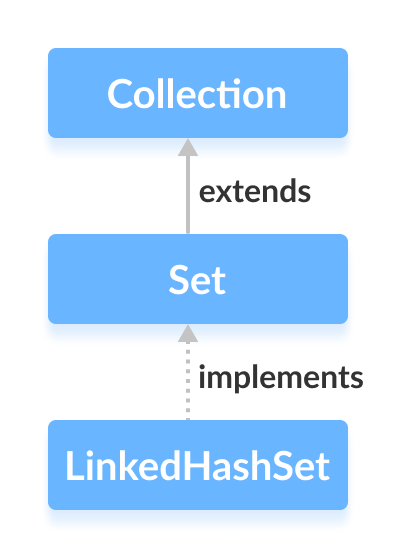The `LinkedHashSet` class of the Java collections framework provides functionalities of both the hashtable and the linked list data structure.

It implements the Set interface.Elements of `LinkedHashSet` are stored in hash tables similar to HashSet.

However, linked hash sets maintain a doubly-linked list internally for all of its elements. The linked list defines the order in which elements are inserted in hash tables.

In order to create a linked hash set, we must import the `java.util.LinkedHashSet` package first.

Once we import the package, here is how we can create linked hash sets in Java.

``````// LinkedHashSet with 8 capacity and 0.75 load factor
``````

Here, we have created a linked hash set named numbers.

Notice, the part `new LinkedHashSet<>(8, 0.75)`. Here, the first parameter is capacity and the second parameter is loadFactor.

• capacity - The capacity of this hash set is 8. Meaning, it can store 8 elements.
• loadFactor - The load factor of this hash set is 0.6. This means, whenever our hash table is filled by 60%, the elements are moved to a new hash table of double the size of the original hash table.

It's possible to create a linked hash set without defining its capacity and load factor. For example,

``````// LinkedHashSet with default capacity and load factor
``````

By default,

• the capacity of the linked hash set will be 16
• the load factor will be 0.75

## Creating LinkedHashSet from Other Collections

Here is how we can create a linked hash set containing all the elements of other collections.

``````import java.util.LinkedHashSet;
import java.util.ArrayList;

class Main {
public static void main(String[] args) {
// Creating an arrayList of even numbers
ArrayList<Integer> evenNumbers = new ArrayList<>();
System.out.println("ArrayList: " + evenNumbers);

// Creating a LinkedHashSet from an ArrayList
}
}

``````

Output

```ArrayList: [2, 4]
```

The `LinkedHashSet` class provides methods that allow us to perform various operations on the linked hash set.

• `add()` - inserts the specified element to the linked hash set
• `addAll()` - inserts all the elements of the specified collection to the linked hash set

For example,

``````import java.util.LinkedHashSet;

class Main {
public static void main(String[] args) {

}
}
``````

Output

```LinkedHashSet: [2, 4, 6]
New LinkedHashSet: [2, 4, 6, 5]
```

To access the elements of a linked hash set, we can use the `iterator()` method. In order to use this method, we must import the `java.util.Iterator` package. For example,

``````import java.util.LinkedHashSet;
import java.util.Iterator;

class Main {
public static void main(String[] args) {

// Calling the iterator() method
Iterator<Integer> iterate = numbers.iterator();

// Accessing elements
while(iterate.hasNext()) {
System.out.print(iterate.next());
System.out.print(", ");
}
}
}
``````

Output

```LinkedHashSet: [2, 5, 6]
LinkedHashSet using Iterator: 2, 5, 6,
```

Note:

• `hasNext()` returns `true` if there is a next element in the linked hash set
• `next()` returns the next element in the linked hash set

## Remove Elements from HashSet

• `remove()` - removes the specified element from the linked hash set
• `removeAll()` - removes all the elements from the linked hash set

For example,

``````import java.util.LinkedHashSet;

class Main {
public static void main(String[] args) {

// Using the remove() method
boolean value1 = numbers.remove(5);
System.out.println("Is 5 removed? " + value1);

boolean value2 = numbers.removeAll(numbers);
System.out.println("Are all elements removed? " + value2);
}
}
``````

Output

```LinkedHashSet: [2, 5, 6]
Is 5 removed? true
Are all elements removed? true
```

## Set Operations

The various methods of the `LinkedHashSet` class can also be used to perform various set operations.

### Union of Sets

Two perform the union between two sets, we can use the `addAll()` method. For example,

``````import java.util.LinkedHashSet;

class Main {
public static void main(String[] args) {

// Union of two set
System.out.println("Union is: " + numbers);
}
}
``````

Output

```LinkedHashSet1: [2, 4]
Union is: [1, 3, 2, 4]
```

### Intersection of Sets

To perform the intersection between two sets, we can use the `retainAll()` method. For example

``````import java.util.LinkedHashSet;

class Main {
public static void main(String[] args) {

// Intersection of two sets
System.out.println("Intersection is: " + evenNumbers);
}
}
``````

Output

```LinkedHashSet1: [2, 3]
Intersection is: 
```

### Difference of Sets

To calculate the difference between the two sets, we can use the `removeAll()` method. For example,

``````import java.util.LinkedHashSet;

class Main {
public static void main(String[] args) {

}
}
``````

Output

```LinkedHashSet1: [2, 3, 5]
Difference: 
```

### Subset

To check if a set is a subset of another set or not, we can use the `containsAll()` method. For example,

``````import java.util.LinkedHashSet;

class Main {
public static void main(String[] args) {

// Check if primeNumbers is a subset of numbers
}
}
``````

Output

```LinkedHashSet1: [1, 2, 3, 4]
```

Method Description
`clone()` Creates a copy of the `LinkedHashSet`
`contains()` Searches the `LinkedHashSet` for the specified element and returns a boolean result
`isEmpty()` Checks if the `LinkedHashSet` is empty
`size()` Returns the size of the `LinkedHashSet`
`clear()` Removes all the elements from the `LinkedHashSet`

To learn more about `LinkedHashSet` methods, visit Java LinkedHashSet (official Java documentation).

Both `LinkedHashSet` and `HashSet` implements the `Set` interface. However, there exist some differences between them.

• `LinkedHashSet` maintains a linked list internally. Due to this, it maintains the insertion order of its elements.
• The `LinkedHashSet` class requires more storage than `HashSet`. This is because `LinkedHashSet` maintains linked lists internally.
• The performance of `LinkedHashSet` is slower than `HashSet`. It is because of linked lists present in `LinkedHashSet`.

Here are the major differences between `LinkedHashSet` and `TreeSet`:
• The `TreeSet` class implements the `SortedSet` interface. That's why elements in a tree set are sorted. However, the `LinkedHashSet` class only maintains the insertion order of its elements.
• A `TreeSet` is usually slower than a `LinkedHashSet`. It is because whenever an element is added to a `TreeSet`, it has to perform the sorting operation.
• `LinkedHashSet` allows the insertion of null values. However, we cannot insert a null value to `TreeSet`.